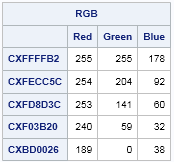In response to my recent post about how to use the PALETTE function in SAS/IML to generate color ramps, a reader wrote the following:

The PALETTE function returns an array of hexadecimal values such as CXF03B20. For those of us who think about colors as RGB values, is there an easy way to convert from hex to RGB?

Yes. In a previous article I explained how to convert from RGB triplets to hexadecimal values. Going the other way is no more difficult. I suggest using the SUBSTR function to extract each pair of hex values. Then use the INPUTN function to apply the HEX2. informat, which converts the hex value to its decimal equivalent. The SAS/IML implementation follows:

```proc iml; /* function to convert an array of colors from hexadecimal to RGB */ start Hex2RGB(_hex); hex = colvec(_hex); /* convert to column vector */ rgb = j(nrow(hex),3); /* allocate three-column matrix for results */ do i = 1 to nrow(hex); /* for each color, translate hex to decimal */ rgb[i,] = inputn(substr(hex[i], {3 5 7}, 2), "HEX2."); end; return( rgb); finish;   /* test the function on a five-color example */ ramp = palette("YLORRD", 5); /* five hex values for SAS colors */ RGB = Hex2RGB(ramp); /* convert to 5 x 3 matrix of RGB values */ print RGB[c={"Red" "Green" "Blue"} r=ramp];```In the DATA step, you can use the same functions to convert hexadecimal colors. However, the SUBSTR function in the DATA step does not accept a vector of positions, so you need to make three separate function calls.

In a 2004 paper, Perry Watts provides a SAS macro called %RGBDec that you can use to convert a hexadecimal color into a string that contains the RGB values.

Share